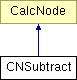# CNSubtract Class Reference

Calculation node subtracting two operand nodes. More...

`#include <expression.h>`

Inheritance diagram for CNSubtract:List of all members.

## Public Member Functions

CNSubtract (CalcNode *_left, CalcNode *_right)
virtual ~CNSubtract ()
virtual double evaluate () const
evaluate the complete calculation tree and return the floating point result value
virtual void print (std::ostream &os, unsigned int depth) const
output the calculation tree to the given stream.

## Private Attributes

CalcNodeleft
left calculation operand
CalcNoderight
right calculation operand

## Detailed Description

Calculation node subtracting two operand nodes.

Definition at line 126 of file expression.h.

## Constructor & Destructor Documentation

 CNSubtract::CNSubtract ( CalcNode * _left, CalcNode * _right ) ` [inline, explicit]`

Definition at line 135 of file expression.h.

 virtual CNSubtract::~CNSubtract ( ) ` [inline, virtual]`

Definition at line 140 of file expression.h.

References left, and right.

## Member Function Documentation

 virtual double CNSubtract::evaluate ( ) const` [inline, virtual]`

evaluate the complete calculation tree and return the floating point result value

Implements CalcNode.

Definition at line 146 of file expression.h.

References CalcNode::evaluate(), left, and right.

 virtual void CNSubtract::print ( std::ostream & os, unsigned int depth ) const` [inline, virtual]`

output the calculation tree to the given stream.

tries to format the output to make tree levels visible.

Implements CalcNode.

Definition at line 151 of file expression.h.

References CalcNode::indent(), left, CalcNode::print(), and right.

## Member Data Documentation

 CalcNode* CNSubtract::left` [private]`

left calculation operand

Definition at line 129 of file expression.h.

Referenced by evaluate(), print(), and ~CNSubtract().

 CalcNode* CNSubtract::right` [private]`

right calculation operand

Definition at line 132 of file expression.h.

Referenced by evaluate(), print(), and ~CNSubtract().

The documentation for this class was generated from the following file:

Generated on Thu Oct 23 11:13:54 2008 for Flex Bison C++ Example by1.5.6